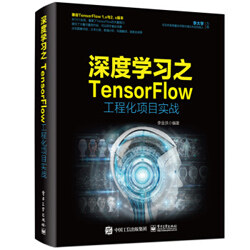# TensorFlow 工程实战（一）：用 TF-Hub 库微调模型评估人物年龄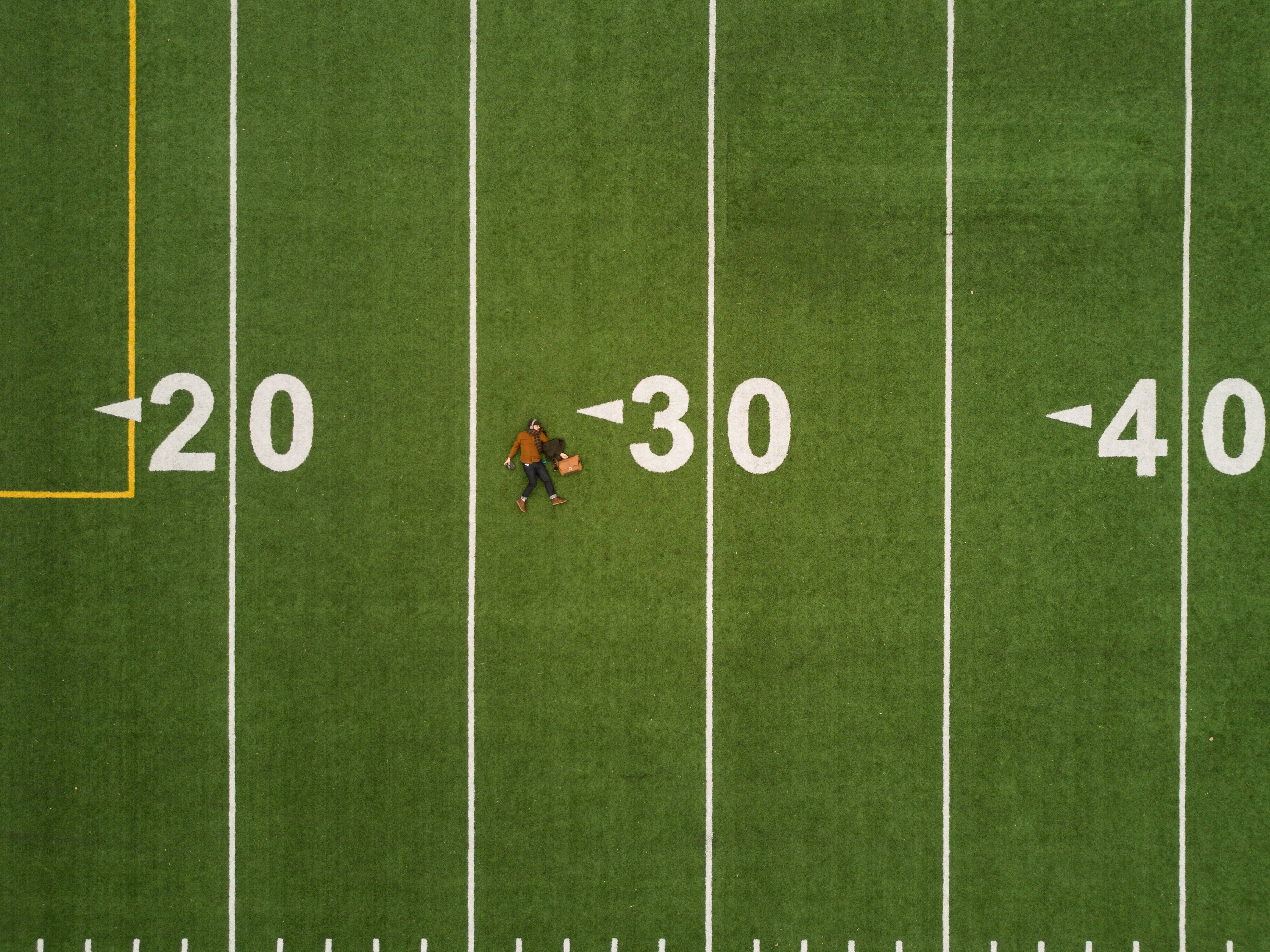## 一、准备样本

• 补充了一些与年龄匹配的人物图片。
• 删掉了若干不合格的样本。

## 二、下载 TF-Hub 库中的模型

### 1. 找到 TF-Hub 库中的模型下载链接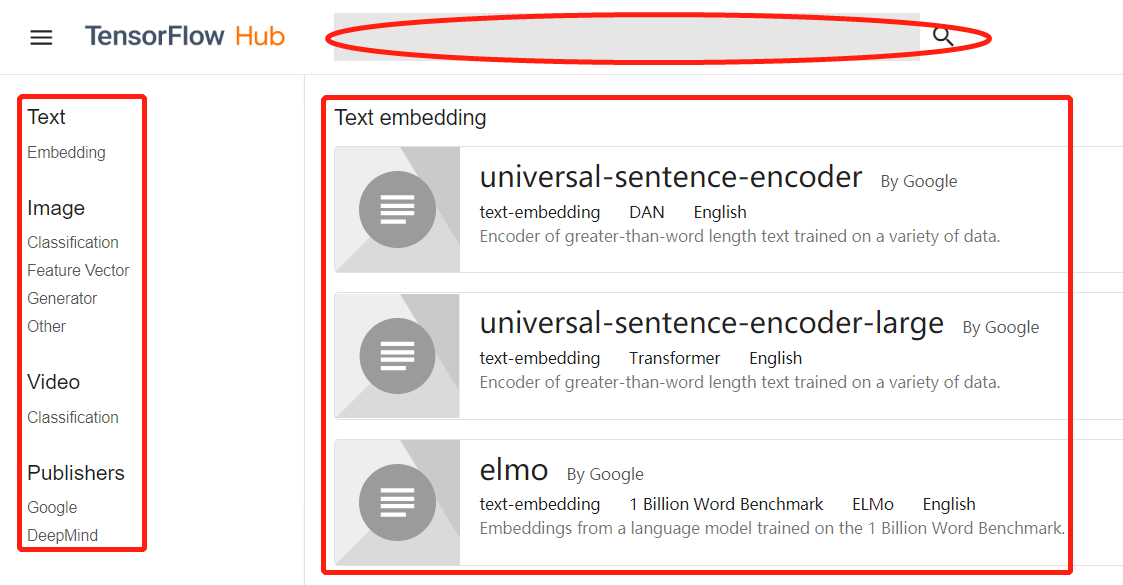• 最顶端是搜索框。可以通过该搜索框搜索想要下载的预训练模型。
• 左侧是模型的分类目录。将 TF-Hub 库中的预训练模型按照文本、图像、视频、发布者进行分类。
• 右侧是具体的模型列表。其中列出每个模型的具体说明和下载链接。

• Classification：是一个分类器模型的分类。该类模型可以直接输出图片的预测结果。用于端到端的使用场景。
• Feature_vector：一个特征向量模型的分类。该类模型是在分类器模型基础上去掉了最后两个网络层，只输出图片的向量特征，以便在预训练时使用。
• Generator：一个生成器模型的分类。该类别的模型可以完成合成图片相关的任务。
• Other：一个有关图像模型的其他分类。

### 2. 在 TF-Hub 库中搜索预训练模型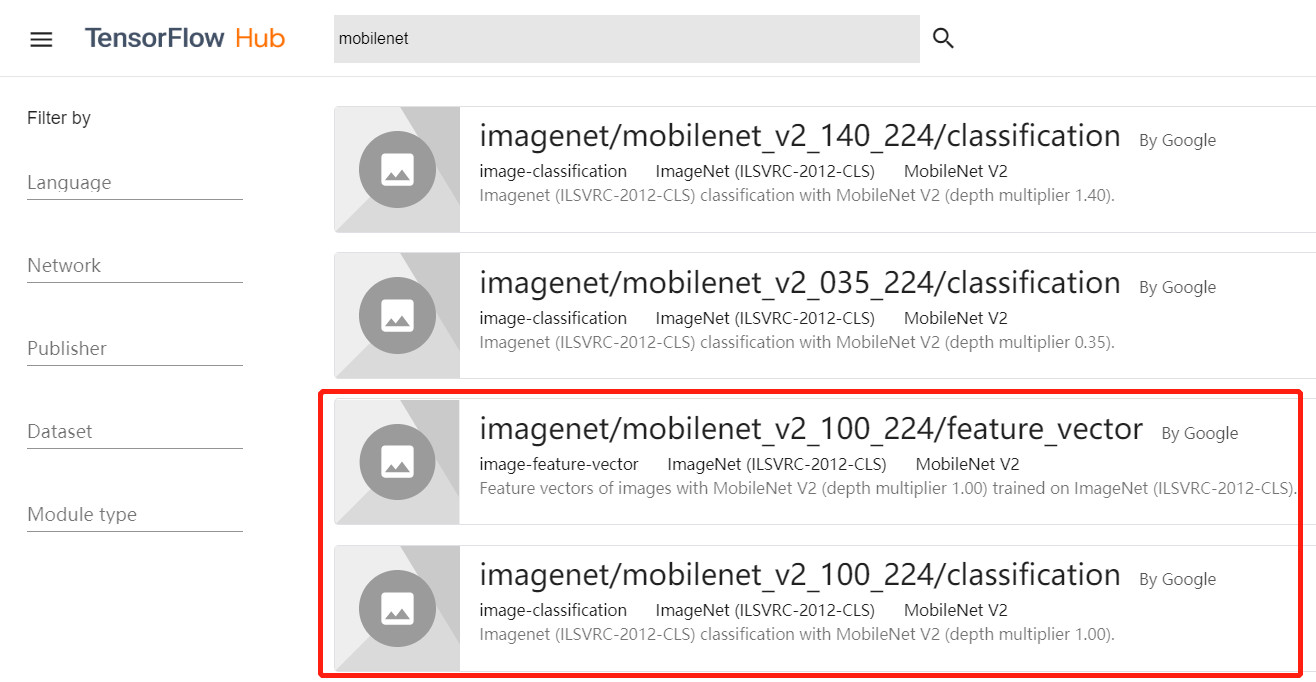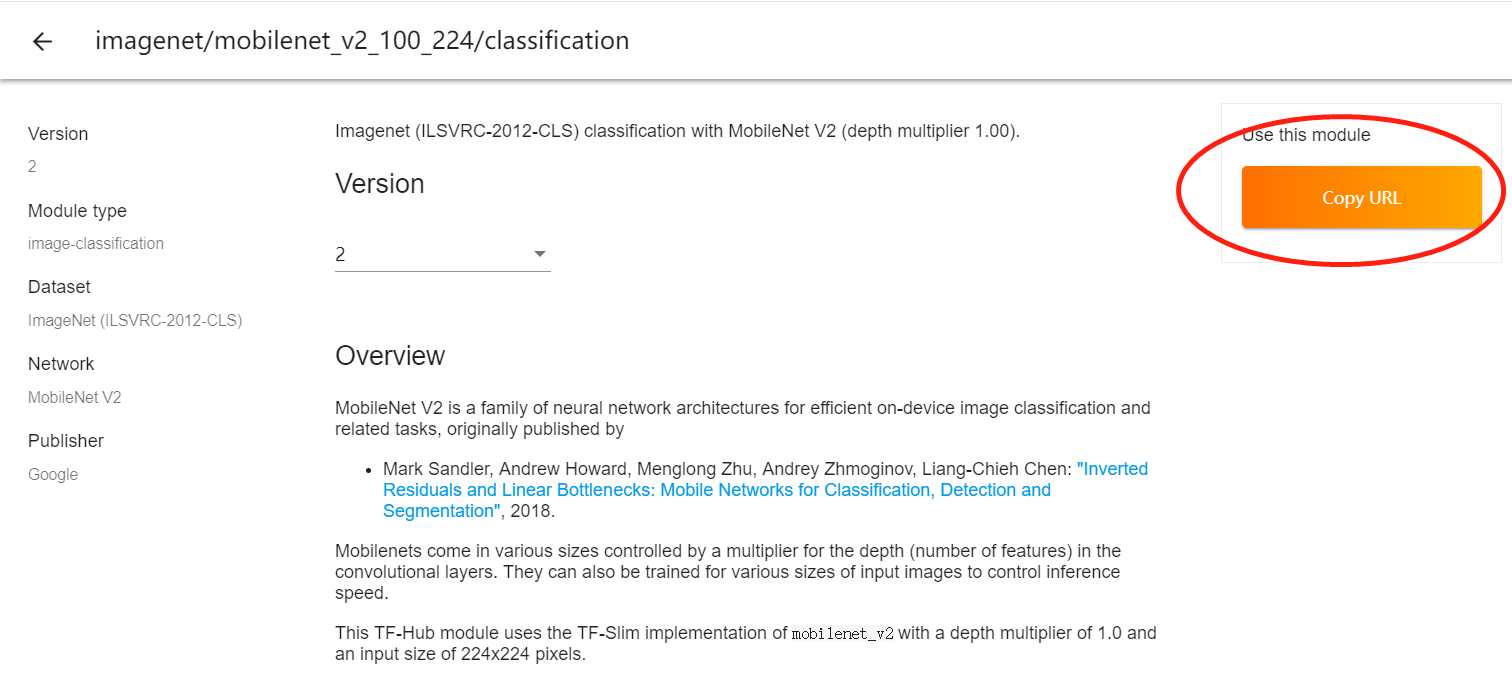### 3. 在 TF-Hub 库中下载 MobileNet_V2 模型

（1）单击 5-8 中的“Copy URL”按钮，所得到的 URL 地址如下：

（3）用下载工具按照（2）中的地址进行下载。

## 三、代码实现：测试 TF-Hub 库中的 MobileNet_V2 模型

from PIL import Imagefrom matplotlib import pyplot as pltimport numpy as npimport tensorflow as tfimport tensorflow_hub as hub with open('中文标签.csv','r+') as f: 				#打开文件			    labels =list( map(lambda x:x.replace(',',' '),list(f))  )    print(len(labels),type(labels),labels[:5]) 		#显示输出中文标签	sample_images = ['hy.jpg', 'ps.jpg','72.jpg']         	#定义待测试图片路径  #加载分类模型  module_spec = hub.load_module_spec("https://tfhub.dev/google/imagenet/mobilenet_v2_100_224/classification/2") #获得模型的输入图片尺寸height, width = hub.get_expected_image_size(module_spec)  input_imgs = tf.placeholder(tf.float32, [None, height,width,3])#定义占位符images = 2 *( input_imgs / 255.0)-1.0                    	#归一化图片 module = hub.Module(module_spec)						#将模型载入张量图 logits = module(images)   #获得输出张量，其形状为 [batch_size, num_classes] y = tf.argmax(logits,axis = 1)                          	#获得结果的输出节点with tf.Session() as sess:    sess.run(tf.global_variables_initializer())    sess.run(tf.tables_initializer())     def preimg(img):                                    	#定义图片预处理函数        return np.asarray(img.resize((height, width)),                           dtype=np.float32).reshape(height, width,3)                               #获得原始图片与预处理图片    batchImg = [ preimg( Image.open(imgfilename) ) for imgfilename in sample_images ]    orgImg = [  Image.open(imgfilename)  for imgfilename in sample_images ]     #将样本输入模型    yv,img_norm = sess.run([y,images], feed_dict={input_imgs: batchImg})        print(yv,np.shape(yv))                                  	#显示输出结果             def showresult(yy,img_norm,img_org):                   #定义显示图片函数        plt.figure()          p1 = plt.subplot(121)        p2 = plt.subplot(122)        p1.imshow(img_org)								#显示图片        p1.axis('off')         p1.set_title("organization image")                p2.imshow((img_norm * 255).astype(np.uint8))		#显示图片        p2.axis('off')         p2.set_title("input image")          plt.show()                print(yy,labels[yy])            for yy,img1,img2 in zip(yv,batchImg,orgImg):          #显示每条结果及图片        showresult(yy,img1,img2)

• 模型是 MobileNet_V2。
• 神经元节点是 100%（无裁剪）。
• 输入的图片尺寸是 224。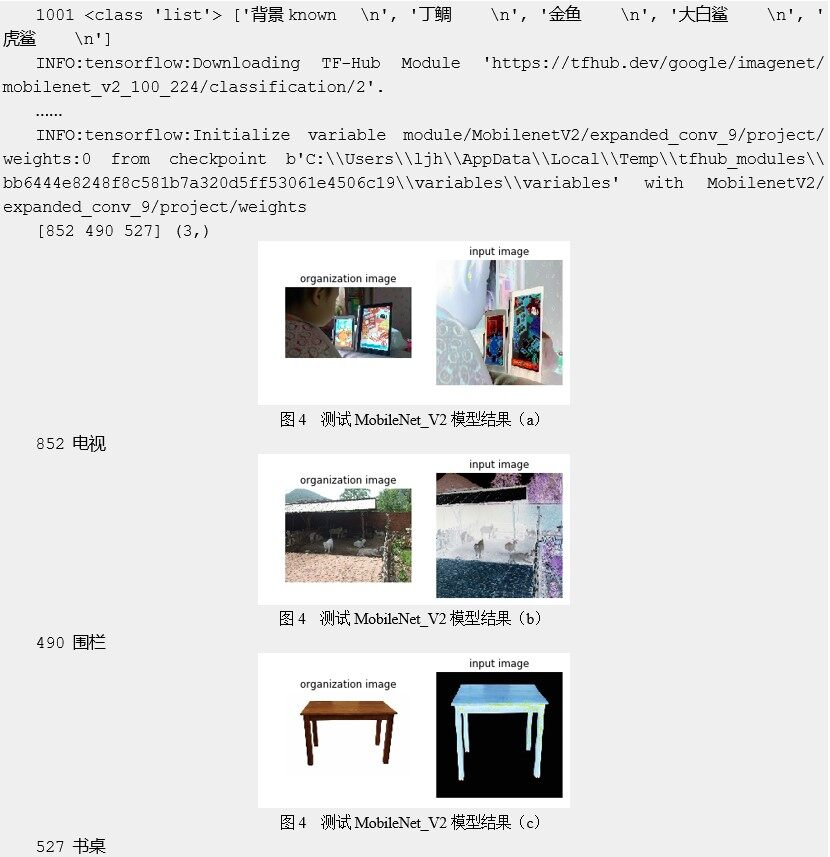• 第 1 行是标签内容。
• 从第 2 行开始，所有以“INFO:”开头的信息都是模型加载具体参数时的日志信息。
在每条信息中都能够看到一个相同的路径：“checkpoint b’C:\Users\ljh\AppData\Local\ Temp\tfhub_modules\bb6444e8248f8c581b7a320d5ff53061e4506c19”，这表示系统将 mobilenet_v2_100_224 模型下载到 C:\Users\ljh\AppData\Local\Temp\tfhub_modules\ bb6444e8248f8c581b7a320d5ff53061e4506c19 目录下。
如果想要让模型缓存到指定的路径下，则需要在系统中设置环境变量 TFHUB_CACHE_DIR。例如，以下语句表示将模型下载到当前目录下的 my_module_cache 文件夹中。

TFHUB_CACHE_DIR=./my_module_cache

module_spec = hub.load_module_spec("mobilenet_v2_100_224")

## 四、用 TF-Hub 库微调 MobileNet_V2 模型

https://github.com/tensorflow/hub/raw/master/examples/image_retraining/retrain.py

### 1. 修改 TF-Hub 库中的代码 BUG

…def get_random_cached_bottlenecks(sess, image_lists, how_many, category,                                 bottleneck_dir, image_dir, jpeg_data_tensor,                                  decoded_image_tensor, resized_input_tensor,                                  bottleneck_tensor, module_name):……  class_count = len(image_lists.keys())  bottlenecks = []  ground_truths = []  filenames = []  if how_many >= 0:    # Retrieve a random sample of bottlenecks.    for unused_i in range(how_many):       IsErr = True         	#添加检测异常标志      while IsErr==True:   	#如果出现异常就再运行一次          try:              label_index = random.randrange(class_count)              label_name = list(image_lists.keys())[label_index]              image_index = random.randrange(MAX_NUM_IMAGES_PER_CLASS + 1)              image_name = get_image_path(image_lists, label_name, image_index,                                      image_dir, category)              IsErr = False 	#没有异常          except ZeroDivisionError:               continue     	   	#出现异常，再运行一次…

### 2. 用命令行运行微调程序

python retrain.py     --image_dir ./IMBD-WIKI   --tfhub_module  mobilenet_v2_100_224_feature_vector

python retrain.py     --image_dir ./IMBD-WIKI  --tfhub_module https://tfhub.dev/google/imagenet/mobilenet_v2_100_224/feature_vector/2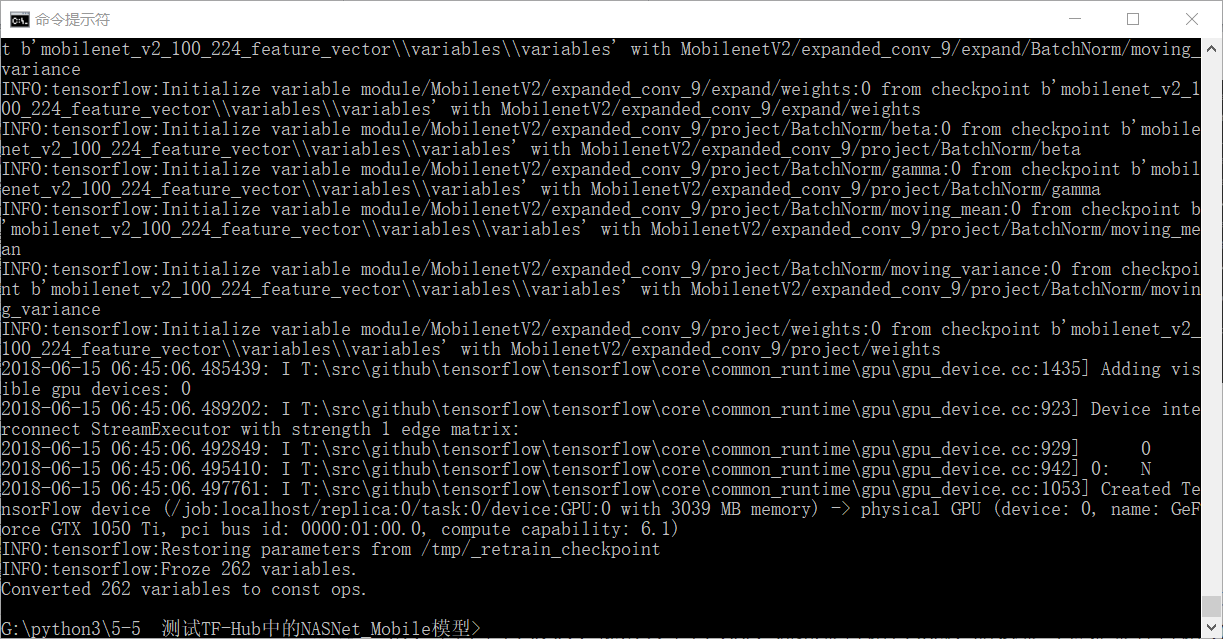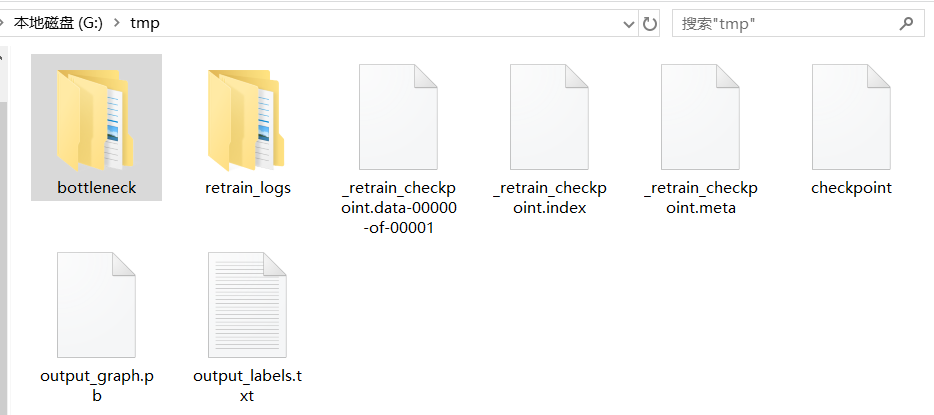• bottleneck：用预训练模型 MobileNet_V2 将图片转化成的特征值文件。
• retrain_logs：微调模型过程中的日志文件。该文件可以通过 TensorBoard 显示出来（TensorBoard 的使用方法见 13.3.2 小节）。

### 3. 支持更多的命令行操作

python  retrain.py     -h

## 五、代码实现：用模型评估人物的年龄

### 1. 找到模型中的输入、输出节点

…def create_module_graph(module_spec):……   height, width = hub.get_expected_image_size(module_spec)  with tf.Graph().as_default() as graph:    resized_input_tensor = tf.placeholder(tf.float32, [None, height, width, 3])    m = hub.Module(module_spec)    bottleneck_tensor = m(resized_input_tensor)    wants_quantization = any(node.op in FAKE_QUANT_OPS                             for node in graph.as_graph_def().node)  return graph, bottleneck_tensor, resized_input_tensor, wants_quantization…

### 2. 加载模型并评估结果

• 样本文件加载部分（见代码第 1~34 行）：这部分重用了本书 4.7 节的代码。
• 加载冻结图（见代码第 35~69 行）：读者可以先有一个概念，在第 13 章还有详细讲解。
• 图片结果显示部分（见代码第 70~94 行）：这部分重用了本书 3.4 节中显示部分的代码。

from PIL import Imagefrom matplotlib import pyplot as pltimport numpy as npimport tensorflow as tf from sklearn.utils import shuffleimport os  def load_sample(sample_dir,shuffleflag = True):    '''递归读取文件。只支持一级。返回文件名、数值标签、数值对应的标签名'''    print ('loading sample  dataset..')    lfilenames = []    labelsnames = []    for (dirpath, dirnames, filenames) in os.walk(sample_dir):               for filename in filenames:                           #遍历所有文件名            #print(dirnames)            filename_path = os.sep.join([dirpath, filename])             lfilenames.append(filename_path)               	#添加文件名            labelsnames.append( dirpath.split('\\')[-1] )#添加文件名对应的标签                lab= list(sorted(set(labelsnames)))  				#生成标签名称列表    labdict=dict( zip( lab  ,list(range(len(lab)))  )) 	#生成字典     labels = [labdict[i] for i in labelsnames]    if shuffleflag == True:        return shuffle(np.asarray( lfilenames),np.asarray( labels)),np.asarray(lab)    else:        return (np.asarray( lfilenames),np.asarray( labels)),np.asarray(lab) #载入标签data_dir = 'IMBD-WIKI\\'  								#定义文件的路径_,labels = load_sample(data_dir,False)   				#载入文件的名称与标签print(labels)							#输出 load_sample 返回的标签字符串 sample_images = ['22.jpg', 'tt2t.jpg']               		#定义待测试图片的路径    tf.logging.set_verbosity(tf.logging.ERROR)tf.reset_default_graph()#分类模型thissavedir= 'tmp'PATH_TO_CKPT = thissavedir +'/output_graph.pb'od_graph_def = tf.GraphDef()with tf.gfile.GFile(PATH_TO_CKPT, 'rb') as fid:    serialized_graph = fid.read()    od_graph_def.ParseFromString(serialized_graph)    tf.import_graph_def(od_graph_def, name='')         fenlei_graph = tf.get_default_graph() height,width = 224,224 with tf.Session(graph=fenlei_graph) as sess:    result = fenlei_graph.get_tensor_by_name('final_result:0')    input_imgs = fenlei_graph.get_tensor_by_name('Placeholder:0')    y = tf.argmax(result,axis = 1)           def preimg(img):                                    		#定义图片的预处理函数        reimg = np.asarray(img.resize((height, width)),                           dtype=np.float32).reshape(height, width,3)         normimg = 2 *( reimg / 255.0)-1.0         return normimg                              #获得原始图片与预处理图片    batchImg = [ preimg( Image.open(imgfilename) ) for imgfilename in sample_images ]    orgImg = [  Image.open(imgfilename)  for imgfilename in sample_images ]        yv = sess.run(y, feed_dict={input_imgs: batchImg})	#输入模型    print(yv)         print(yv,np.shape(yv))                                  	#显示输出结果             def showresult(yy,img_norm,img_org):                   #定义显示图片的函数        plt.figure()          p1 = plt.subplot(121)        p2 = plt.subplot(122)        p1.imshow(img_org)								#显示图片        p1.axis('off')         p1.set_title("organization image")                img = ((img_norm+1)/2)*255        p2.imshow(  np.asarray(img,np.uint8)      )		#显示图片        p2.axis('off')         p2.set_title("input image")              plt.show()                print(" 索引：",yy,","," 年纪：",labels[yy])            for yy,img1,img2 in zip(yv,batchImg,orgImg):        	#显示每条结果及图片        showresult(yy,img1,img2)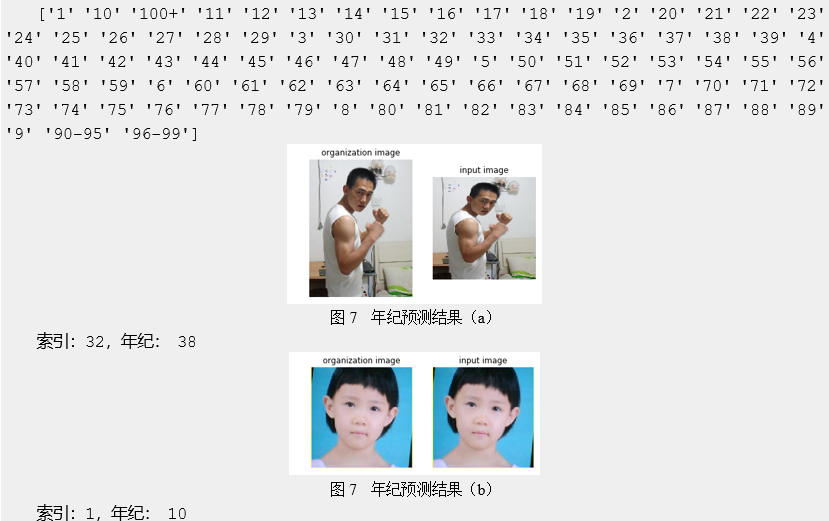• 第 1 部分是标签的内容。
• 第 2 部分是评估的结果。